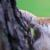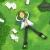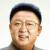linux字符串替换问题，有点难度

bt21cn 发布于 2015/07/07 18:49

(1,1,{1,1},{1,1,{2,2},cco1{2,2},cbax[{2,2},{2,2}]},{ccp1[{2,2},{2,2}]})

(1,1,{1,1},{1,1,{2|2},cco1{2|2}|cbax[{2|2}|{2|2}]},{ccp1[{2|2}|{2|2}]})

0sed 's/{2\,2\}/\{2\|2\}/g'
b
2这个值只是我举的例子，为了方便查看， 实际情况是不同的值
0
b
ding ding
0b

0i = 0
M = 0
len = length string

for (;;)
if i >= len
break

c = string[i++]

if c == ,
if M == 2
c = |
if c == {
M++
if c == }
if M > 0
M--b